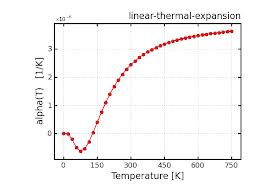Wednesday, 25 September 2013

Is CoE Important?

CoE is more important to the manufacturer (in combination with viscosity) than to the kiln worker. It has gained a heightened profile, as it has been used as a shorthand for compatibility. So it is important to know what CoE is and what the numbers mean.

During heat transfer, the energy that is stored in the intermolecular bonds between atoms changes. When the stored energy increases, so does the length of the molecular bond. As a result, solids typically expand in response to heating and contract on cooling; this response to temperature change is expressed as its coefficient of … expansion.
The ... expansion coefficient is a thermodynamic property of a substance. It relates the change in temperature to the change in a material's linear dimensions. It is the fractional change in length [metres] per degree [C] of temperature change [expressed as a two digit whole number].
Most solids expand when heated. The reason for this is that this gives atoms more room to bounce about with the large amount of kinetic energy they have at high temperatures. Thermal expansion is a relatively small effect which is approximately linear in the [absolute] temperature range.”

What does CoE mean?

There are at least two types of expansion with increasing temperature. One is volume expansion and the other that we are more interested in, is the linear expansion. “The Coefficient of Linear Expansion of a substance is the fraction of its original length by which a rod [or sheet] of the substance expands per degree rise in temperature.” Source

What do the numbers mean?

The numbers attached to a CoLE -usually referred to as CoE – are an expression of the average amount that a material expands per degree over a given temperature range. The standard temperature range is 0ºC to 300ºC and the unit of length is one metre. They are expressed as a two digit number to the power of -6. That means the two digit number really has 6 decimal points in front of the whole number. So a CoLE of 85 means the same as an expansion rate of .000085 metres per degree C; or .0085mm/ºC.

However the rate of expansion is not a straight line when graphed against higher temperatures. The ranges in which kiln formers work show an erratic and much higher rate of expansion. Have a look at the CoE ranges at different temperatures to see how variable the expansion rates are at elevated temperatures.  Other examples are:Graph showing the change in the CoLE of aluminium between 0ºC and 527ºC (Kelvin being about 273 degrees lower than Celsius)This graph shows a material that actually contracts briefly as it warms.  Its CoLE would be between 20 and 35 - an extremely low rate of expansion.This shows an idealised material that has a CoLE of  about 40 at 0ºC and around 60 at 300ºC, remaining thereabouts as the temperature rises toward 1200ºC

Should We use CoE?

CoLE is “a meaningless number unless defined by the temperature range in which the measurement is taken. Calling any glass or glass combination “compatible” without specifying under what conditions is no more useful than identifying a glass by its COE without specifying the relevant temperature range.” [L. MacGreggor]